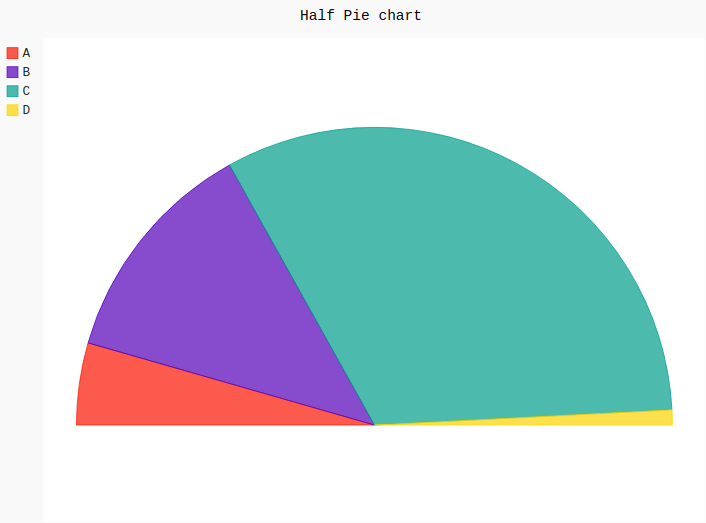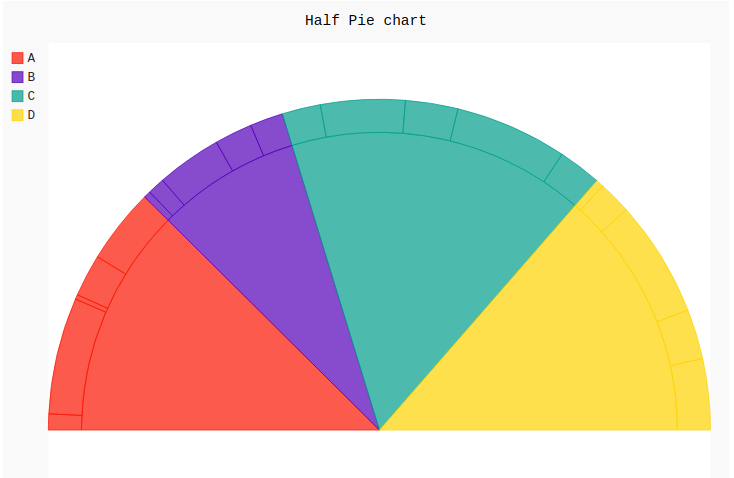# Half pie chart in Pygal

Pygal is a Python module that is mainly used to build SVG (Scalar Vector Graphics) graphs and charts. SVG is a vector-based graphics in the XML format that can be edited in any editor. Pygal can create graphs with minimal lines of code that can be easy to understand.

## Half Pie Chart

A half pie chart is also known as half-moon or semi-circle pie chart which is a 180-degree graph that represents the anatomy of a whole pie. The half pie chart works similarly to the regular pie chart. Its main advantage is that it takes less space in the layout and works like a full pie chart does. All the areas are plotted with different colors which helps to understand it more efficiently. It can be created using the `Pie()` method of pygal module by passing the half_pie attribute. This takes a boolean value.

Syntax:

`pie_chart = pygal.Pie(half_pie=True)`

Example 1:

 `# importing pygal ` `import` `pygal ` ` `  ` `  `# creating line chart object ` `pie_chart ``=` `pygal.Pie(half_pie ``=` `True``) ` ` `  `# naming the title ` `pie_chart.title ``=` `'Half Pie chart'` ` `  ` `  `# random data ` `pie_chart.add(``'A'``, ``115``) ` `pie_chart.add(``'B'``, ``322``) ` `pie_chart.add(``'C'``, ``834``) ` `pie_chart.add(``'D'``, ``21``) ` ` `  `pie_chart `

Output:Example 2:

 `# importing pygal ` `import` `pygal ` `import` `numpy ` ` `  ` `  `# creating line chart object ` `pie_chart ``=` `pygal.Pie(half_pie ``=` `True``) ` ` `  `# naming the title ` `pie_chart.title ``=` `'Half Pie chart'` ` `  ` `  `# Random data ` `pie_chart.add(``'A'``, numpy.random.rand(``5``)) ` `pie_chart.add(``'B'``, numpy.random.rand(``5``)) ` `pie_chart.add(``'C'``, numpy.random.rand(``5``)) ` `pie_chart.add(``'D'``, numpy.random.rand(``5``)) ` ` `  `pie_chart `

Output:Attention geek! Strengthen your foundations with the Python Programming Foundation Course and learn the basics.

To begin with, your interview preparations Enhance your Data Structures concepts with the Python DS Course.

My Personal Notes arrow_drop_upIf you like GeeksforGeeks and would like to contribute, you can also write an article using contribute.geeksforgeeks.org or mail your article to contribute@geeksforgeeks.org. See your article appearing on the GeeksforGeeks main page and help other Geeks.

Please Improve this article if you find anything incorrect by clicking on the "Improve Article" button below.

Article Tags :

Be the First to upvote.

Please write to us at contribute@geeksforgeeks.org to report any issue with the above content.